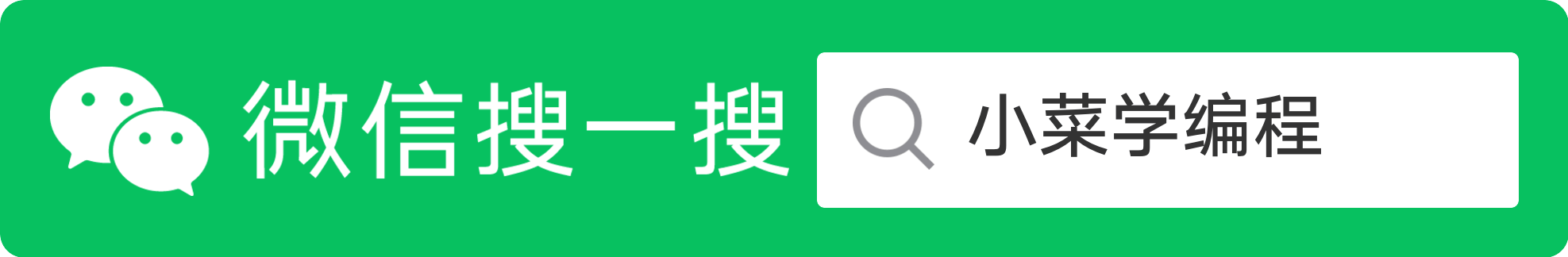# Go语言基本类型

Go 内置了以下基本类型：

• 布尔
• bool
• 字符串
• string
• 整数
• int int8 int16 int32 int64
• uint uint8 uint16 uint32 uint64
• 字节
• byteuint8 的别名
• Unicode
• runeint32 的别名
• 浮点
• float32 float64
• 复数
• complex64 complex128

  1 2 3 4 5 6 7 8 9 10 11 12 13 14 15 16 17 18  package main import ( "fmt" "math/cmplx" ) var ( ToBe bool = false MaxInt uint64 = 1 << 64 -1 z complex128 = cmplx.Sqrt(-5 +12i) ) func main() { fmt.Printf("Type: %T Value: %v\n", ToBe, ToBe) fmt.Printf("Type: %T Value: %v\n", MaxInt, MaxInt) fmt.Printf("Type: %T Value: %v\n", z, z) } 

## 零值

• 对于数值类型是 0
• 对于布尔类型是 false
• 对于字符串类型是 "" （空字符串）；
  1 2 3 4 5 6 7 8 9 10 11  package main import "fmt" func main() { var i int var f float64 var b bool var s string fmt.Printf("%v %v %v %q\n", i, f, b, s) } 

## 类型转换

 1 2 3  var i int = 42 var f float64 = float64(i) var u uint = uint(f) 

 1 2 3  i := 42 f := float(i) u := uint(f) 

C 语言有所不同， Go 在不同类型之间赋值，需要显式类型转换。 不信，将下面例子中 float64unit 类型转换移除，看看发生什么？

  1 2 3 4 5 6 7 8 9 10 11 12 13  ackage main import ( "fmt" "math" ) func main() { var x, y int = 3, 4 var f float64 = math.Sqrt(float64(x*x + y*y)) var z uint = uint(f) fmt.Println(x, y, z) } 

## 类型推断

 1 2  var i int j := i // j is an int as well 

 1 2 3  i := 42 // int f := 3.142 // float64 g := 0.867 + 0.5i // complex128 

 1 2 3 4 5 6 7 8  package main import "fmt" func main() { v := 42 // change me! fmt.Printf("v is of type %T\n", v) } 

【小菜学Go语言】系列文章首发于公众号【小菜学编程】，敬请关注：【小菜学Go语言】系列文章首发于公众号【小菜学编程】，敬请关注：